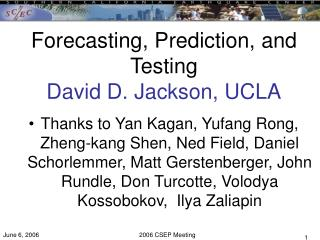# Forecasting, Prediction, and Testing David D. Jackson, UCLA - PowerPoint PPT PresentationDownload PresentationForecasting, Prediction, and Testing David D. Jackson, UCLA

Forecasting, Prediction, and Testing David D. Jackson, UCLA
Download Presentation## Forecasting, Prediction, and Testing David D. Jackson, UCLA

- - - - - - - - - - - - - - - - - - - - - - - - - - - E N D - - - - - - - - - - - - - - - - - - - - - - - - - - -
##### Presentation Transcript

1. Forecasting, Prediction, and TestingDavid D. Jackson, UCLA • Thanks to Yan Kagan, Yufang Rong, Zheng-kang Shen, Ned Field, Daniel Schorlemmer, Matt Gerstenberger, John Rundle, Don Turcotte, Volodya Kossobokov, Ilya Zaliapin

2. Definitions • Forecast: specification of the probability per unit area, magnitude, time, focal mechanism, etc. • Prediction: special case of forecasting in which the probability in some region is much higher than normal, and high enough to justify exceptional . • Notes: a prediction must be temporary; it also requires a definition of normal (i.e., a null hypothesis).

3. Why forecast and test • Test hypotheses of earthquake physics • Quasiperiodic characteristic earthquakes • Magnitudes limited by fault geometry • Moment balance (tectonic in = seismic out) • Earthquake rate proportional to stress rate • Inform decisions about earthquake risk • Facility locations, building codes, insurance rates, retrofit, etc. • Inspire envy

4. Some testable statements • At least one event will occur within given region, time interval, and magnitude interval with probability p. • For many small intervals, this is RELM type forecast • Replace region by “segment” and have WG88 type forecast. But “earthquake on segment” is subjective. • N events will occur within given region, time interval, and magnitude interval with probability p(N). • The largest event in given region and time interval will be m, with probability p(m). My recommendation for a usable and stable forecast. • If an event occurs in region 1, time interval 1, and magnitude interval 1, it will be in the included region 2, time interval 2, and magnitude interval 2 with probability p. (KB type forecast) • The next earthquake in a given region and magnitude interval will not be before time t with probability p(t). Replace region by “segment” and have WG88 type forecast.

5. What we can and can’t do now • Can • Forecast 90% of quakes in 10% of area. • Predict aftershocks • Forecast mag-freq relationship for N(m)>10. • Maybe can • Forecast earthquake rate from deformation rate. • Establish earthquake rate for “normal” conditions • Forecast Mmax • Can’t • Predict times of individual earthquakes

6. Likelihood Testing • Simulate catalogs using L(lat,lon) • Compute log likelihood function for observed and simulated catalogs • Sort L values in increasing order, plot order vs L.

7. Practical problems in testing • Many users: many criteria • Earthquakes not independent • Testing should be rigorous, but also “feel good.” • Data on rupture lengths, endpoints of rupture, slip distribution, etc., not formalized

8. Ten-year Prospective Test of Seismic Gap and Null (Poissonian Smoothed Seismicity) models Rank zones by decreasing probability of characteristic earthquake; accumulate area, predicted earthquake number, and actual earthquake number.

9. Properties of test methods • Likelihood ratio test • Includes absolute rates • Useful for marked point process: lat, lon, mag, etc. • As used, assumes independent events; • Adaptable to time varying forecasts • Could use declustered catalog • Gives scalar “score” • Likelihood ratio test on largest event in zone, time interval • Reduces effect of dependent events • May respond to long-term users: investors, planners • Molchan diagram • Based on relative rates, as normally used • Needs a scalar measure to reject a hypothesis • Not convenient for marked point processes; need to commit to magnitude threshold, e.g. • Does not avoid problem of dependent events

10. Testing scheme for elements of risk model

11. Conclusions • Many users => Many criteria for optimality • Absolute rate vs. relative rate • Short time vs. long time • Big events vs. smaller ones • Biggest problem is interdependence of quakes • Long term users want stability: unconditional probabilities • Scientists care about interactions: conditional probabilities • Solutions to interdependence • Decluster catalog: needs model • Test using conditional probabilities updated automatically • Test only largest earthquake in zone.